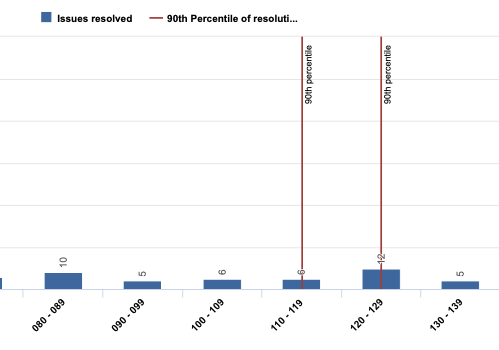# CASE logic returning unexpected results for Monte Carlo chart percentile line

Hello, I am trying to build a Monte Carlo style chart based off of Resolution Interval dimension and using Issues resolved measure. It mostly works, but I get an unexpected result. I am trying to show a vertical line at the range matching 90th percentile. I am getting 2 hits on my CASE, when I expect only one. For reference, my 90th percentile value is 124 so I expect the line to land between 120 - 129 range.CASE WHEN
– left part of range is less than percentile…
– extract the first element of the range using regex
Val(ExtractString([Resolution interval].CurrentMember.Name,
“(\d+) - (\d+)”,1)) <
Percentile(
Filter(Descendants([Issue].CurrentMember,[Issue].[Issue]),
([Measures].[Issues resolved],
[Resolution interval].DefaultMember)>0
),
([Measures].[total resolution days],
[Resolution interval].DefaultMember),
90
)
AND
– and…right part of range is greater than percentile
– extract the second element of the range
Val(ExtractString([Resolution interval].CurrentMember.NextMember.Name,
“(\d+) - (\d+)”,2)) >

Percentile(
Filter(Descendants([Issue].CurrentMember,[Issue].[Issue]),
([Measures].[Issues resolved],
[Resolution interval].DefaultMember)>0
),
([Measures].[total resolution days],
[Resolution interval].DefaultMember),
90
)

THEN
– call out the percentile at the range
“90th percentile”

END

Hi @Ryan_Kent,

I checked the calculation and in the second condition, where you take the next interval name (`[Resolution interval].CurrentMember.NextMember.Name`), you extract the second part of the name, effectively comparing that the number is between this member start and next member end. Please try to change the second part of the check to the following:

``````...
AND
-- and…right part of range is greater than percentile
-- extract the second element of the range
Val(ExtractString([Resolution interval].CurrentMember.NextMember.Name,
"(\d+) - (\d+)",1)) >

Percentile(
...
``````

Lauma / support@eazybi.com

Thank you @lauma.cirule! I missed that it was NextMember for the second part. This makes sense to me and works as expected now. Appreciate your help!

1 Like